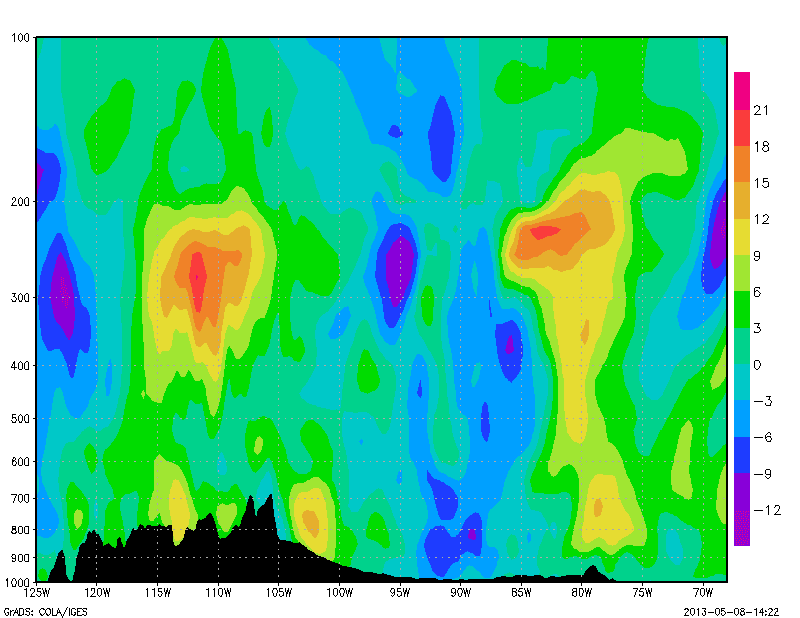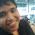## Wednesday, May 8, 2013

### Tutorial: Vertical Cross section with topography in pressure coordinates

Vertical cross sections are very easy to plot in GrADS, requiring only that you fix either the latitude or longitude value, and vary the height levels.  For example, an west to east cross section is easily set up using the following commands.

'set lat 40.0'
'set lon -125 -68'
'set lev 1000 100'

A lot of cross sections however like to add in the topography, giving the cross section greater structure.  The method to do this in GrADS is not terribly hard or difficult, it is just a little tricky.

The first step is to fix your page area using the 'set parea' command.  This is because the in order to overlay topography onto your cross section, we need to change around the domain, but keep domains plotting in the same spot.  Setting the page area assures this.  Once your page are is set, just plot your desired cross section variable as usual.

Now it's time to overlay the topography.  In pressure coordinates, the topography can be represented by the surface pressure (surface pressure decreases with increased topographical height).  Since the surface pressure is a 2D and not a 3D variable, you have to fix the height in GrADS to the lowest model level (usually done by 'set lev 1000', or 'set z 1').  Now that you have done this, you need to fix the y-axis of the plot to match the y-axis of your cross section.  This is done with the 'set vrange' command.  The best way to do this is to set it exactly as you would have set the levs when plotting your cross section variable.

For example, if you typed in 'set lev 1000 100' for your cross section then you would type in 'set vrange 1000 100' for your topography.

This way, your topography is set to plot on the same scale as your cross section.  Now, we are ready to plot.  To do this, we make use of the 'set gxout linefill' option.  Then we simply fill the gap between the surface pressure and the baseline pressure of 1000.  The block of code to do this looks is shown below.

'set lev 1000'
'set vrange 1000 100'
'set gxout linefill'
'set lfcols 1 1'
'd pressfc/100;const(pressfc/100,1000)'

The 'set lfcols' command is the syntax for setting the color of the topography.  The end result for an east west cross-section through the United States will look something like this:Example of Cross section with topography

An additional way to plot topography is by using the 'set gxout bar' command, instead of the 'set gxout linefill' command.  The syntax is similar, but with minor differences.  You set the vrange the same, but instead of having to fill between two values, you now only need to plot the variable.  Visually, this gives your topography a more discrete, blocky look to it.

Syntax for plotting topography with 'set gxout bar':

'set gxout bar'
'set baropts filled'
'set ccolor 1'
'set lev 1000'
'set vrange 1000 100'
'd pressfc/100'

Resultant Image:

The real difficulty comes with rying to plot a vertical cross section on a log scale.  Since surface pressure is only a 2D variable, it is unaffected when setting the y-axis to be on a log scale.  As a result, neither of the above methods are capable of correctly plotting the topography on a log scale.  So in order to do this, we need to essentially trick GrADS into plotting the topography using a different method.

This is a little tricky to explain, but the basic idea is you simply plot the geopotential height at contour intervals so close together that they overlap and appear as one entity.  Since we are focused on topography, we subtract the surface geopotential height (elevation) from the geopotential height.  Also, since we are only interested in plotting the topography, any value >0 is masked out.  Hopefully, this short paragraph gives you some indication of what this next block of code is doing.

'set gxout contour'
'set clab off'
size=750
int=10
i=0
'hgt=hgtprs-hgtsfc'

while(i*size>=-5000)
j=i-1
'set ccolor 1'
'set cthick 10'
'set cstyle 1'
'set cmax 'i*size
'set cmin 'j*size
'set cint 'int
'd hgt'
i=i-1
endwhile

Now that you can look at the code, I can explain it a little more thoroughly.  Basically, we need a while loop because GrADS cannot set enough contour levels at one time, so we have to continuously reset the contour levels until we cover the full range of values.  Within the loop we reset the line thickness and the line style to ensure continuity through the plotting.

The result of this code is a correctly plotted vertical cross section on a log scale with topography.Vertical cross section (with terrain) plotted on a log scale

Hopefully this tutorial has provided you with a greater understanding of how to plot vertical cross sections in GrADS (at least in pressure coordinates).

1.Hi there,
Thank you for providing such a great tutorial. Actually, I'm trying to follow your tutorial to plot a proper vertical cross-section with topography by using WRF outputs. I did the first part of your tutorial, but for second part (adding log scale) I really confused which variable of WRF outputs can be used for producing such a cross section instead of geopotential height (my WRF output doesn't have geopotential height as a variable). I would greatly appreciate it if you kindly provide me an example with WRF outputs. Thanks, Abbas

2.Are you using the WRF output interpolated to pressure coordinates? or are you using the sigma coordinate output? Also, if you have some topography variable, or surface pressure, I have a good work around for you.

3.Thanks for your prompt response. I'm using WRF output with pressure coordinates. Here are some variables might be helpful:
height 27 0 Model height (KM)
pressure 27 0 Model pressure (hPa)
psfc 1 0 surface pressure (Pa)

1.can anyone tell me which command we need to follow for plotting the levels height

4.Okay, so the first thing to check would be trying to do this with the height variable (presuming you have a topography variable), this method would (should) work if you subtracted topography from the model height (be sure the units match up).

If that doesn't work, we can fudge it using surface pressure in a more complicated manner. The method used is the same one used for doing arbitrary vertical cross sections (tutorial provided below).

I'll let you get the specifics on how to get a terrain shading using surface pressure from the tutorial but basically, what you will do is first plot your data using the log scale, and loop through each longitude value (or grid-point), and grab the surface pressure value (use 'set gxout print' to print it to the screen -- you should only have one value per grid point, and be sure you get it into the same units as the model pressure on the y-axis) Once you have the surface pressure saved, run the 'q w2xy' command to find out this locations 'page coordinates', and then save the x/y coordinates into an array (I included a link to my tutorial on arrays also). Then once you loop through all grid points, just draw a filled polygon using your array ('draw polyf 'array). That should draw your terrain in log coordinates using only a surface variable.
I know that's fairly complicated, especially if you are new to GrADS, but it does work, and maybe first try using the method here using the model height variable, it's been a while since I worked directly with WRF output in GrADS, but you should have a topography variable in there somewhere.

Best of luck!

5.Hello,

Thank you for the great tutorial. I did a little experiment with GrADS, and I found the following codes gave similar result :

# First part for plotting the meridional wind cross section without topography in log scale
ga-> set parea 1 10 1 7.5
ga-> set lev 1000 100
ga-> set zlog on
ga-> d vgrdprs

# Second part for plotting the topography in log scale. It's almost similar with yours, but I added 'set logd' for log scaling since surface pressure is 1-layer variable.
ga-> set lev 1000
ga-> set vrange 1000 100
ga-> set log1d logv
ga-> set gxout linefill
ga-> set lfcols 1 1
ga-> d pressfc/100;const(pressfc/100,1000)

Would you check it. Thanks :-)

6.hello,
i want to plot pressure from 1000 to 50 in y axis and time from 0 to 24 in x axis, and i want to display reflectivity. I done the following steps but i dont know how to set pressure so that we get pressure on y axis, please help me to set pressure , Thank you.